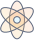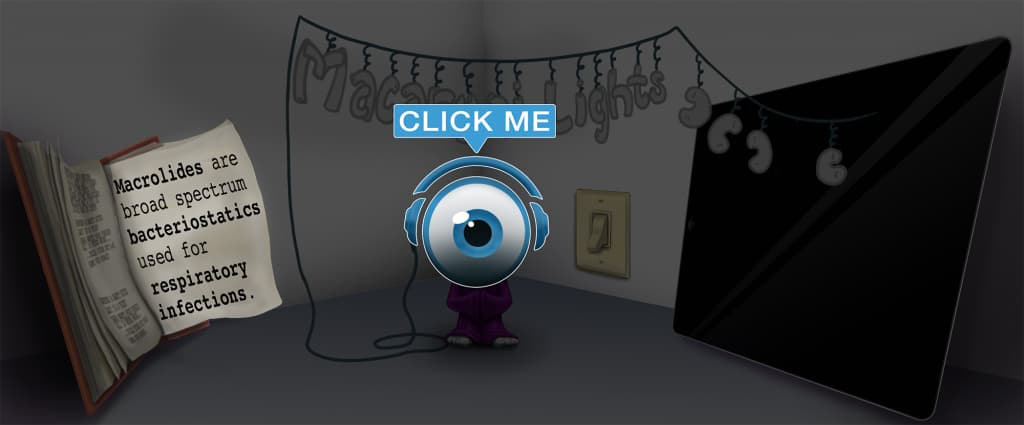# Master Metric System with Picmonic for Nursing RN

## With Picmonic, facts become pictures. We've taken what the science shows - image mnemonics work - but we've boosted the effectiveness by building and associating memorable characters, interesting audio stories, and built-in quizzing.

### Metric System

Metro SystemPicmonic
Health professionals use the metric system, so it is important to be familiar with it for appropriate dosing calculations and administration. In the metric system, weight is measured in units of grams; as we commonly see prescriptions written in grams and milligrams. 1 kilogram (kg) = 1,000 grams (g), 1 gram (g) = 1,000 milligrams (mg), 1 milligram (mg) = 1,000 micrograms (mcg), and 1000 micrograms (mcg) = 1 milligram (mg). In the metric system, the liter (L) is used to describe volume. One liter = 1000 milliliters (mL). The meter is used to describe length in the metric system. Conversions between the units in the metric system can be done by multiplying or dividing by 1000. To convert larger to smaller, multiply by 1,000, or move the decimal point 3 places to the right. To convert smaller to larger, divide by 1,000, or move the decimal point 3 spaces to the left.
9 KEY FACTS
WEIGHT
Kilogram (kg)
Killa-graham-cracker

In the metric system,the gram (g/gm) is used to describe weight. 1 kilogram (kg)= 1,000 grams (g).

Gram (g/gm)
Graham-cracker

In the metric system, the gram (g/gm) is used to describe weight. 1 gram (g) = 1,000 milligrams (mg).

Milligram (mg)
Millionaire-graham-cracker

In the metric system, the gram (g/gm) is used to describe weight. 1 milligram (mg) = 1,000 mcg.

Microgram (mcg)
Microphone-graham-cracker

In the metric system, the gram (g/gm) is used to describe weight. 1,000 microgram (mcg) = 1 milligram (mg).

VOLUME
Liter (L)
Liter-of-cola

In the metric system, the liter (L) is used to describe volume.1 liter = 1,000 milliliters (mL).

Milliliter (mL)
Millionaire-liter-of-cola

In the metric system, the liter (L) is used to describe volume. 1 liter = 1,000 milliliters (mL).

LENGTH
Meter (m)
Meter-Pan

In the metric system, the meter is used to describe length.

CONVERTING
Larger to Smaller: Multiply
Large Metro Car Turned into a Small Metro Car by Multiplying

The metric system applies the idea that units within the system get larger or smaller by a power of 10. Conversions between the units in the metric system can be done by multiplying or dividing by 1,000. To convert larger to smaller, multiply by 1,000, or move the decimal point 3 places to the right.

Smaller to Larger: Divide
Small Metro Car Turned into a Large Metro Car by Dividing

The metric system applies the idea that units within the system get larger or smaller by a power of 10. Conversions between the units in the metric system can be done by multiplying or dividing by 1,000. To convert smaller to larger, divide by 1,000 or move the decimal point 3 spaces to the left.

Unlock all 9 facts & definitions with Picmonic Free!

## Take the Metric System Quiz

Picmonic's rapid review multiple-choice quiz allows you to assess your knowledge.

## Picmonic for Nursing RN CoversRegistered Nurse (RN)
1,100+ Videos
13,000+ Facts

## Our Story Mnemonics Increase Mastery and Retention

Memorize facts with phonetic mnemonics

Unforgettable characters with concise but impactful videos (2-4 min each)

### Ace YourRegistered Nurse (RN)Classes & Exams with Picmonic:

#### Over 1,200,000 students use Picmonic’s picture mnemonics to improve knowledge, retention, and exam performance.